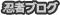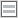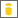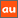# 0除算に関するアナウンスメントを公開しています

The purpose of this site is to publish announcements on the theory of division by zero

## Announcement 293: Parallel lines on the Euclidean plane from the viewpoint of division by zero $1/0=0$

Institute of Reproducing Kernels
Kawauchi-cho, 5-1648-16,
Kiryu 376-0041, Japan
E-mail: kbdmm360@yahoo.co.jp
March 27, 2016

Abstract: In this announcement, for its importance we would like to declare that any parallel lines have the common point $(0,0)$ in the sense of the division by zero.  From this fact we have to change our basic idea for the Euclidean plane and  we will see a new world for not only mathematics, but also the universe.

Introduction
By a natural extension of the fractions
\begin{equation}
\frac{b}{a} \tag{0.1}
\end{equation}for any complex numbers $a$ and $b$, we found the result, for any complex number $b$
\begin{equation}
\frac{b}{0}=0, \tag{0.2}
\end{equation}incidentally in  by the Tikhonov regularization for the Hadamard product inversions for matrices, and we discussed their properties and gave several physical interpretations on the general fractions in  for the case of real numbers. The result is a very special case for general fractional functions in .
The division by zero has a long and mysterious story over the world $($see, for example, Google site with division by zero$)$ with its physical viewpoints since the document of zero in India on AD 628, however, Sin-Ei, Takahasi  $($$)$ $($see also  $)$ established a simple and decisive interpretation $($1.2$)$ by analyzing some full extensions of fractions and by showing the complete characterization for the property $($1.2$)$:

Proposition. Let F be a function from ${\bf C }\times {\bf C }$ to ${\bf C }$ satisfying
\begin{equation}
F (b, a)F (c, d)= F (bc, ad)
\end{equation}for all $a, b, c, d \in {\bf C }$, and
$$F (b, a) = \frac {b}{a }, \quad a, b \in {\bf C }, a \ne 0.$$Then, we obtain, for any $b \in {\bf C }$
$F (b, 0) = 0.$
We thus should consider, for any complex number $b$, as  $($1.2$)$;
that is, for the mapping
\begin{equation}
w = \frac{1}{z},
\end{equation}
the image of $z=0$ is $w=0$ $($should be defined$)$. This fact seems to be a curious one in connection with our well-established popular image for the  point at infinity on the Riemann sphere $($$)$. Therefore, the division by zero will give great impacts to complex analysis and to our ideas for the space and universe.

However, the division by zero $($1.2$)$ is now clear, indeed, for the introduction of $($1.2$)$, we have several independent approaches as in:
1) by the generalization of the fractions by the Tikhonov regularization or by the Moore-Penrose generalized inverse,
2) by the intuitive meaning of the fractions $($division$)$ by H. Michiwaki,
3) by the unique extension of the fractions by S. Takahasi,   as in the above,
4) by the extension of the fundamental function $W = 1/z$ from ${\bf C} \setminus \{0\}$ into ${\bf C}$ such that $W =1/z$ is a one to one and onto mapping from ${\bf C} \setminus \{0\}$ onto ${\bf C} \setminus \{0\}$ and the division by zero $1/0=0$ is a one to one and onto mapping extension of the function $W =1/z$ from  ${\bf C}$ onto ${\bf C}$,
and
5) by considering the values of functions with the mean values of functions.
Furthermore, in $($$)$ we gave the results in order to show the reality of the division by zero in our world:
A) a field structure  containing the division by zero --- the Yamada field ${\bf Y}$,
B)  by the gradient of the $y$ axis on the $(x,y)$ plane --- $\tan \frac{\pi}{2} =0$,
C) by the reflection $1/\overline{z}$ of $z$ with respect to the unit circle with center at the origin on the complex $z$ plane --- the reflection point of zero is zero,
and
D) by considering rotation of a right circle cone having some very interesting
phenomenon  from some practical and physical problem --- EM radius.
See also  for the relationship between fields and the division by zero, and the importance of the division by zero for computer science.

Meanwhile,  J. P.  Barukcic and I.  Barukcic $($$)$ discussed recently the relation between the division $0/0$ and special relativity of Einstein.  Furthermore,  Reis and Anderson $($[8, 9]$)$ extends the system of the real numbers by defining division by zero. Meanwhile, we should refer to up-to-date information:
Kurt Arbenz  https://www.researchgate.net/publication/272022137  Riemann Hypothesis Addendum -   Breakthrough.
Here, we recall Albert Einstein’s words on mathematics: Blackholes are where God divided by zero. I don't believe in mathematics. George Gamow $($1904-1968$)$ Russian-born American nuclear physicist and cosmologist remarked that ”it is well known to students of high school algebra” that divi-sion by zero is not valid; and Einstein admitted it as the biggest blunder of his life  1. Gamow, G., My World Line $($Viking, New York$)$. p 44, 1970.
For our results, see the survey style announcements 179, 185, 237, 246, 247, 250 and 252 of the Institute of Reproducing Kernels $($[13, 14, 15, 16, 17, 18, 19]$)$.
At this moment, the following theorem may be looked as the fundamental theorem of the division by zero:

Theorem (). Any analytic function takes a definite value at an isolated singular point with a natural meaning.

The following corollary shows how to determine the value of an analytic function at the singular point; that is, the value is determined from the regular part of the Laurent expansion:

Corollary. For an isolated singular point $a$ of an analytic function $f(z)$, we have the Cauchy integral formula$$f(a) = \frac{1}{2\pi i} \int_{\gamma} f(z) \frac{dz}{z - a},$$where the $\gamma$ is a rectifiable simple Jordan closed curve that surrounds one time the point $a$ on a regular region of the function $f(z)$.

The essential meaning of this theorem and corollary is given by that:  the values of functions may be understood in the sense of the mean values of analytic functions.

In this announcement, we will state the basic property of parallel lines by the division by zero on the Euclidean plane and we will be able to see that the division by zero introduces a new world and  fundamental mathematics.
In particular, note that the concept of parallel lines is very important in the Euclidean plane and non-Euclidean geometry. The essential results may be stated as known since the discovery of the division by zero  $z/0=0$. However, for importance, we would like to state clearly  the details.

The point at infinity
We will be able to see the whole Euclidean plane by the stereographic projection  into the Riemann sphere --- We think that in the Euclidean plane, there does not exist the point at infinity. However, we can consider it as a limit like $\infty$. Recall the definition of $z \to \infty$ by $\epsilon$-$\delta$ logic; that is,  $\lim_{z \to \infty} z = \infty$ if and only if for any large $M>0$, there exists a number $L>0$ such that for any z satisfying $L <|z|$, $M<|z|$. In this definition,  the infinity $\infty$ does not appear. The infinity is not a number, but it is an ideal space point.
The behavior of the space around the point at infinity may be considered by that around the origin by the linear transform $W = 1/z$ $($$)$. We thus see that
\begin{equation}
\lim_{z \to \infty} z = \infty,\tag{2.1}
\end{equation}however,\begin{equation}
[z]_{z =\infty} =0,\tag{2.2}
\end{equation} by the division by zero. The difference of $($2.1$)$ and $($2.2$)$ is very important as we see clearly by the function $1/z$ and the behavior at the origin. The limiting value to the origin and the value at the origin are different. For  surprising results, we will state the property in the real space as follows:
\begin{equation}
\lim_{x\to  +\infty} x =+\infty , \quad \lim_{x\to  -\infty} x = -\infty,\tag{2.3}
\end{equation} however
\begin{equation}
[x]_{ +\infty } =0, \quad  [x]_{ -\infty } =0.\tag{2.4}
\end{equation}
Interpretation by analytic geometry
We write lines by
\begin{equation}
L_k:  a_k x + b_k y  + c_k = 0, k=1,2. \tag{3.1}
\end{equation} The common point is given by, if $a_1 b_2 - a_2 b_1 \ne 0$; that is, the lines are not parallel
\begin{equation}
\left(\frac{b_1 c_2 -  b_2 c_1}{a_1 b_2 - a_2 b_1}, \frac{a_2 c_1 - a_1 c_2}{a_1 b_2 - a_2 b_1}\right).\tag{3.2}
\end{equation} By the division by zero, we can understand that if $a_1 b_2 - a_2 b_1 = 0$, then the commom point is always given by
\begin{equation}
(0,0),\tag{3.3}
\end{equation}even the two lines are the same. This fact shows that the image of the Euclidean space in Section 2 is right.

Remarks
For a function
\begin{equation}
S(x,y) = a(x^2+y^2) + 2gx + 2fy + c,\tag{4.1}
\end{equation}the radius $R$ of the circle $S(x,y) = 0$ is given by
\begin{equation}
R  = \sqrt{\frac{g^2 +f^2 -ac}{a^2}}.\tag{4.2}
\end{equation}If $a = 0$, then the area $\pi R^2$ of the circle is zero, by the division by zero; that is, the circle is line $($degenerate$)$.
Here, note that by the Theorem, $R^2$ is zero for $a = 0$, but for (4.2) itself
\begin{equation}
R  = \frac{-c}{2} \frac{1}{\sqrt{g^2  + f^2}}\tag{4.3}
\end{equation}for $a=0$. However, this result will be nonsense, and so, in this case, we should consider $R$ as zero as $0^2 =0$. When we apply the division by zero to functions, we can consider, in general, many ways.

For example, for the function $z/(z-1)$, when we insert $z=1$  in numerator and denominator, we have\begin{equation}
\left[\frac{z}{z-1}\right]_{z = 1} = \frac{1}{0} =0.\tag{4.4}
\end{equation}However, in the sense of the Theorem,
from the identity\begin{equation}
\frac{z}{z-1} = \frac{1}{z-1} + 1,\tag{4.5}
\end{equation}we have\begin{equation}
\left[\frac{z}{z-1}\right]_{z = 1} = 1.\tag{4.6}
\end{equation} By the Theorem, for analytic functions we can give uniquely determined values at isolated singular points, however, the values by means of the Laurent expansion are not always reasonable. We will need to consider many interpretations for reasonable values.
In addition, the center of the circle (4.3) is given by\begin{equation}
\left( - \frac{g}{a},- \frac{f}{a}\right).\tag{4.7}
\end{equation}Therefore, the center of a general line
\begin{equation}
2gx + 2fy + c=0\tag{4.8}
\end{equation}may be considered as the origin $(0,0)$,  by the division by zero.
We can see similarly  the 3 dimensional versions. We consider the functions\begin{equation}
S_j(x,y) = a_j(x^2+y^2) + 2g_jx + 2f_jy + c_j.\tag{4.9}
\end{equation}The distance $d$ of the centers of the circles $S_1(x,y) =0$ and $S_2(x,y) =0$ is given by\begin{equation}
d^2= \frac{g_1^2 + f_1^2}{a_1^2} - 2 \frac{g_1 g_2 + f_1 f_2}{a_1 a_2} +  \frac{g_2^2 + f_2^2}{a_2^2}.\tag{4.10}
\end{equation}If $a_1 =0$, then by the division by zero
\begin{equation}d^2=    \frac{g_2^2 + f_2^2}{a_2^2}.\tag{4.11}
\end{equation}Then,  $S_1(x,y) =0$ is a line and its center is the origin $(0,0)$.

References
 L. V. Ahlfors, Complex Analysis, McGraw-Hill Book Company, 1966.
 J. P.  Barukcic and I.  Barukcic, Anti Aristotle - The Division Of Zero By Zero,
ViXra.org $($Friday, June 5, 2015$)$ © Ilija Barukčić, Jever, Germany. All rights reserved. Friday, June 5, 2015 20:44:59.
 J. A. Bergstra, Y. Hirshfeld and J. V. Tucker, Meadows and the equational specification of division $($arXiv:0901.0823v1[math.RA] 7 Jan 2009$)$.
 L. P.  Castro and S. Saitoh,  Fractional functions and their representations,  Complex Anal. Oper. Theory 7 $($2013$)$, no. 4, 1049-1063.
 M. Kuroda, H. Michiwaki, S. Saitoh, and M. Yamane,
New meanings of the division by zero and interpretations on $100/0=0$ and on $0/0=0$,
Int. J. Appl. Math.  27 $($2014$)$, no 2, pp. 191-198,  DOI: 10.12732/ijam.v27i2.9.
 H. Michiwaki, S. Saitoh,  and  M.Yamada, Reality of the division by zero $z/0=0$.  IJAPM International J. of Applied Physics and Math. 6 $($2015$)$, 1--8.　http://www.ijapm.org/show-63-504-1.html
 H. Michiwaki, S. Saitoh and M. Takagi,  A new concept for the point at infinity and the division by zero z/0=0 $($manuscript$)$.
 T. S. Reis and James A.D.W. Anderson, Transdifferential and Transintegral Calculus,
Proceedings of the World Congress on Engineering and Computer Science 2014 Vol I
WCECS 2014, 22-24 October, 2014, San Francisco, USA
 T. S. Reis and James A.D.W. Anderson, Transreal Calculus, IAENG  International J. of Applied Math., 45:  IJAM 45 10 6.
 S. Saitoh, Generalized inversions of Hadamard and tensor products for matrices,  Advances in Linear Algebra & Matrix Theory.  4  $($2014$)$, no. 2,  87--95. http://www.scirp.org/journal/ALAMT/
 S.-E. Takahasi,  On the identities $100/0 = 0$ and $0/0 = 0$. $($note$)$
 S.-E. Takahasi, M. Tsukada and Y. Kobayashi,  Classification of continuous fractional binary operations on the real and complex fields,  Tokyo Journal of Mathematics, 38 $($2015$)$, no. 2, 369-380.
 Announcement 179 $($2014.8.30$)$: Division by zero is clear as z/0=0 and it is fundamental in mathematics.
 Announcement 185 $($2014.10.22$)$: The importance of the division by zero $z/0=0$.
 Announcement 237 $($2015.6.18$)$:  A reality of the division by zero $z/0=0$ by  geometrical optics.
 Announcement 246 $($2015.9.17$)$: An interpretation of the division by zero $1/0=0$ by the gradients of lines.
 Announcement 247 $($2015.9.22$)$: The gradient of y-axis is zero and $\tan (\pi/2) =0$ by the division by zero $1/0=0$.
 Announcement 250 $($2015.10.20$)$: What are numbers? -  the Yamada field containing the division by zero $z/0=0$.
 Announcement 252 $($2015.11.1$)$: Circles and curvature - an interpretation by Mr. Hiroshi Michiwaki of the division by zero $r/0 = 0$.
 Announcement 281$($2016.2.1$)$, The importance of the division by zero $z/0=0$.
 Announcement 282$($2016.2.2$)$, The division by zero $z/0=0$ on the Second Birthday.

PR

### コメント

お名前 Black LimeGreen SeaGreen Teal FireBrick Tomato IndianRed BurlyWood SlateGray DarkSlateBlue LightPink DeepPink DarkOrange Gold DimGray Silverプロフィール

ＨＮ：

(01/17)
(01/14)
(01/12)
(01/04)
(01/03)